## Union

The union of two setsand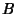is the set obtained by combining the members of each. This is written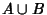, and is pronouncedunion'' orcup.'' The union of sets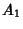through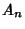is written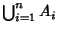.

Let,,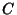, ... be sets, and let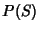denote the probability of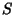. Then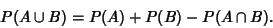(1)

Similarly,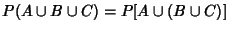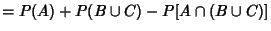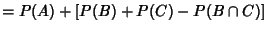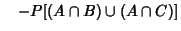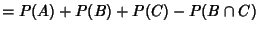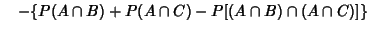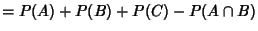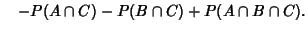(2)
Ifandare Disjoint, by definition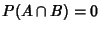, so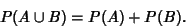(3)

Continuing, for a set ofdisjoint elements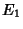,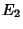, ...,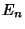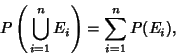(4)

which is the Countable Additivity Probability Axiom. Now let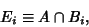(5)

then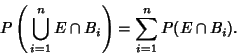(6)